# 鱼眼镜头的畸变纠正分析The Analysis of Fish-Eye Lens Distortion Calibration

• 全文下载: PDF(828KB)    PP.139-149   DOI: 10.12677/GST.2019.73020
• 下载量: 344  浏览量: 1,361

The ultra-wide-angle lens’ focal length is 16 mm or shorter, and the angle of view is close to or equal to 180 degrees, commonly known as “fish-eye lens”. In order to make the lens reach the maximum viewing angle, the front lens of this lens is short in diameter and parabolic, projecting toward the front of the lens, which is similar to the fish’s eye, so “fish-eye lens” is named. Fish-eye lens has a large measurement range, but also large distortion, so it is not widely used in photogrammetry. If there is an effective correction method for the fish-eye lens image, it can be used in some measurements. Nowadays, the study of digital camera calibration is already quite mature, and the photogrammetry and computer vision experts both at home and abroad put forward many kinds of effective calibration method. Although there is a lot of camera calibrations method, to the fish-eye lens it is still immature. In this paper, I will focus on analyzing and comparing the existing fish-eye lens calibration models for fish-eye lens and achieve the calibration algorithm by programming, and finally use the stereoscopic measurement way to illustrate the accuracy of fish-eye lens calibration.

1. 引言

2. 研究趋势

3. 标定模型

3.1. 经典标定模型

$\begin{array}{l}x+\Delta x-{x}_{0}=-{C}_{i}\frac{\left\{{m}_{11}\left(X-{X}_{0}\right)+{m}_{12}\left(Y-{Y}_{0}\right)+{m}_{13}\left(Z-{Z}_{0}\right)\right\}}{\left\{{m}_{31}\left(X-{X}_{0}\right)+{m}_{32}\left(Y-{Y}_{0}\right)+{m}_{33}\left(Z-{Z}_{0}\right)\right\}}\\ y+\Delta y-{y}_{0}=-{C}_{j}\frac{\left\{{m}_{11}\left(X-{X}_{0}\right)+{m}_{12}\left(Y-{Y}_{0}\right)+{m}_{13}\left(Z-{Z}_{0}\right)\right\}}{\left\{{m}_{31}\left(X-{X}_{0}\right)+{m}_{32}\left(Y-{Y}_{0}\right)+{m}_{33}\left(Z-{Z}_{0}\right)\right\}}\end{array}$ (1)

$\begin{array}{l}x=\frac{{L}_{1}X+{L}_{2}Y+{L}_{3}}{{L}_{7}X+{L}_{8}Y+1}\\ y=\frac{{L}_{4}X+{L}_{5}Y+{L}_{6}}{{L}_{7}X+{L}_{8}Y+1}\end{array}$ (2)

$\begin{array}{l}\Delta x=\left(x-{x}_{0}\right)\left({k}_{1}{r}^{2}+{k}_{2}{r}^{4}+\cdots \right)+{p}_{1}\left({r}^{2}+2{\left(x-{x}_{0}\right)}^{2}\right)+2{p}_{2}\left(x-{x}_{0}\right)\left(y-{y}_{0}\right)\\ \Delta y=\left(y-{y}_{0}\right)\left({k}_{1}{r}^{2}+{k}_{2}{r}^{4}+\cdots \right)+{p}_{2}\left({r}^{2}+2{\left(y-{y}_{0}\right)}^{2}\right)+2{p}_{1}\left(x-{x}_{0}\right)\left(y-{y}_{0}\right)\end{array}$ (3)

3.2. 鱼眼相机成像模型

i) 中心投影： $r=f×\mathrm{tan}\left(\theta \right)$ (4)

ii) 立体投影： $r=2×f×\mathrm{tan}\left(\theta /2\right)$ (5)

iii) 等距投影： $r=f×\theta$ (6)

iv) 等角投影： $r=2×f×\mathrm{sin}\left(\theta /2\right)$ (7)

v) 正交投影： $r=f×\mathrm{sin}\left(\theta \right)$ (8)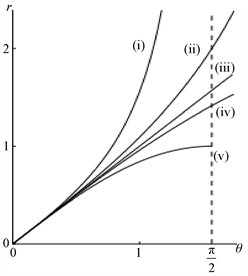Figure 1. Functional relationship between projection radius r and incident angle theta in the above five projection models

$r={k}_{1}×\theta +{k}_{2}×{\theta }^{2}+{k}_{3}×{\theta }^{3}+{k}_{4}×{\theta }^{4}+{k}_{5}×{\theta }^{5}+\cdots +{k}_{n}×{\theta }^{n}$ (9)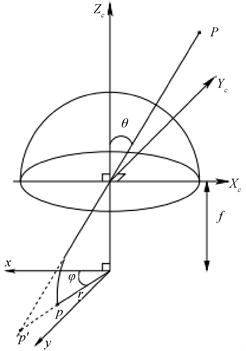Figure 2. Diagram of the relationship between projection radius and incident angle in projection model of fish-eye camera

$u=r\left(\theta \right)×\mathrm{cos}\left(\phi \right)×mu+{u}_{0}$ (15)

$v=r\left(\theta \right)+\mathrm{sin}\left(\phi \right)×mv+{v}_{0}$ (16)

4. 实验过程

4.1. 特征点提取

1) 对原图进行中值滤波，然后用Canny算子提取边缘，如图3所示。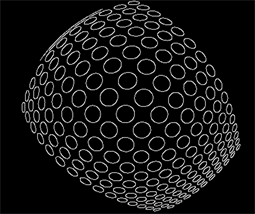Figure 3. Edge binary images obtained by canny operator

2) 人工选择区域并且由计算机将识别圆心，如图4所示。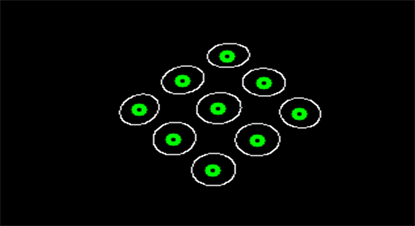Figure 4. Artificially selected area and automatically recognized by computer (green ring)

4.2. 内参数初始化

$\begin{array}{l}{u}_{0}=\frac{width}{2},\\ {v}_{0}=\frac{height}{2},\end{array}$ (17)

(17)式中： $widht$ 为影像以像素为单位的宽度， $height$ 为影像以像素为单位的高度，对于 $mu,mv$ ，可以由像片大小，视场角和焦距算出，式中符号含义与前文相同

$\begin{array}{l}mu=\frac{width}{2}/\left(f×\theta \right)\\ mv=\frac{height}{2}/\left(f×\theta \right)\end{array}$ (18)

4.3. 外参数初始化

$\begin{array}{l}x=\frac{{h}_{1}X+{h}_{2}Y+{h}_{3}}{{h}_{7}X+{h}_{8}Y+1}\\ y=\frac{{h}_{4}X+{h}_{5}Y+{h}_{6}}{{h}_{7}X+{h}_{8}Y+1}\end{array}$ (19)

(X,Y)为控制点坐标，(x,y)为相应的像点坐标，当控制点大于等于4个时，将式(18)线性化，通过线性方程求解二维dlt的8个参数，由于8个参数是内外方为元素的函数值，由这8个参数解出外方位元素。当平面控制点的z坐标为0时，经典的共线方程可表示为式(19)。

$\begin{array}{l}x-{x}_{0}=-f\frac{{a}_{1}\left(X-{X}_{s}\right)+{b}_{1}\left(Y-{Y}_{S}\right)+{c}_{1}\left(-{Z}_{s}\right)}{{a}_{3}\left(X-{X}_{s}\right)+{b}_{3}\left(Y-{Y}_{S}\right)+{c}_{3}\left(-{Z}_{s}\right)}\\ y-{y}_{0}=-f\frac{{a}_{2}\left(X-{X}_{s}\right)+{b}_{2}\left(Y-{Y}_{S}\right)+{c}_{2}\left(-{Z}_{s}\right)}{{a}_{3}\left(X-{X}_{s}\right)+{b}_{3}\left(Y-{Y}_{S}\right)+{c}_{3}\left(-{Z}_{s}\right)}\end{array}$ (20)

$\begin{array}{l}x=\frac{\left(f\frac{{a}_{1}}{\lambda }-\frac{{a}_{3}}{\lambda }{x}_{0}\right)X+\left(f\frac{{b}_{1}}{\lambda }-\frac{{b}_{3}}{\lambda }{x}_{0}\right)Y+\left({x}_{0}-\frac{f}{\lambda }\left({a}_{1}{X}_{s}+{b}_{1}{Y}_{s}+{c}_{1}{Z}_{s}\right)\right)}{-\frac{{a}_{3}}{\lambda }X-\frac{{b}_{3}}{\lambda }Y+1}\\ y=\frac{\left(f\frac{{a}_{2}}{\lambda }-\frac{{a}_{3}}{\lambda }{y}_{0}\right)X+\left(f\frac{{b}_{2}}{\lambda }-\frac{{b}_{3}}{\lambda }{y}_{0}\right)Y+\left({y}_{0}-\frac{f}{\lambda }\left({a}_{2}{X}_{s}+{b}_{2}{Y}_{s}+{c}_{2}{Z}_{s}\right)\right)}{-\frac{{a}_{3}}{\lambda }X-\frac{{b}_{3}}{\lambda }Y+1}\end{array}$ (21)

$\begin{array}{l}{h}_{1}=f\frac{{a}_{1}}{\lambda }-\frac{{a}_{3}}{\lambda }{x}_{0}\\ {h}_{2}=f\frac{{b}_{1}}{\lambda }-\frac{{b}_{3}}{\lambda }{x}_{0}\\ {h}_{3}={x}_{0}-\frac{f}{\lambda }\left({a}_{1}{X}_{S}+{b}_{1}{Y}_{S}+{c}_{1}{Z}_{s}\right)\\ {h}_{4}=f\frac{{a}_{1}}{\lambda }-\frac{{a}_{3}}{\lambda }{y}_{0}\\ {h}_{5}=f\frac{{b}_{2}}{\lambda }-\frac{{b}_{3}}{\lambda }{y}_{0}\\ {h}_{6}={y}_{0}-\frac{f}{\lambda }\left({a}_{2}{X}_{S}+{b}_{2}{Y}_{S}+{c}_{2}{Z}_{s}\right)\\ {h}_{7}=-\frac{{a}_{3}}{\lambda }\\ {h}_{8}=-\frac{{b}_{3}}{\lambda }\end{array}\right\}$ (22)

$\begin{array}{l}\frac{{h}_{1}-{h}_{7}{x}_{0}}{f}=\frac{{a}_{1}}{\lambda }\\ \frac{{h}_{2}-{h}_{8}{x}_{0}}{f}=\frac{{b}_{1}}{\lambda }\\ \frac{{h}_{4}-{h}_{7}{y}_{0}}{f}=\frac{{a}_{2}}{\lambda }\\ \frac{{h}_{5}-{h}_{8}{y}_{0}}{f}=\frac{{b}_{2}}{\lambda }\\ -{h}_{7}=-\frac{{a}_{3}}{\lambda }\\ -{h}_{8}=-\frac{{b}_{3}}{\lambda }\end{array}\right\}$ (23)

$\begin{array}{l}\frac{{a}_{1}}{{a}_{3}}=-\frac{{h}_{1}-{h}_{7}{x}_{0}}{fh{}_{7}}\\ \frac{{b}_{1}}{{b}_{3}}=-\frac{{h}_{2}-{h}_{8}{x}_{0}}{fh{}_{8}}\\ \frac{{a}_{2}}{{a}_{3}}=-\frac{{h}_{4}-{h}_{7}{y}_{0}}{fh{}_{7}}\\ \frac{{b}_{2}}{{b}_{3}}=-\frac{{h}_{5}-{h}_{8}{y}_{0}}{fh{}_{8}}\end{array}\right\}$ (24)

${b}_{1}^{2}+{b}_{2}^{2}+{b}_{3}^{2}=1$

${b}_{3}^{2}=\frac{1}{1+\frac{{\left({h}_{2}-{h}_{8}{x}_{0}\right)}^{2}}{{f}^{2}{h}_{8}^{2}}+\frac{{\left({h}_{5}-{h}_{8}{y}_{0}\right)}^{2}}{{f}^{2}{h}_{8}^{2}}}$ (25)

$\left(\begin{array}{c}{c}_{1}\\ {c}_{2}\\ {c}_{3}\end{array}\right)=\left(\begin{array}{c}{a}_{1}\\ {a}_{2}\\ {a}_{3}\end{array}\right)×\left(\begin{array}{c}{b}_{1}\\ {b}_{2}\\ {b}_{3}\end{array}\right)=\left(\begin{array}{c}{a}_{2}{b}_{3}-{a}_{3}{b}_{2}\\ {a}_{3}{b}_{1}-{a}_{1}{b}_{3}\\ {a}_{1}{b}_{2}-{a}_{2}{b}_{1}\end{array}\right)$ (26)

$\mathrm{tan}\left(\varphi \right)=-\frac{{a}_{3}}{{c}_{3}}=\frac{1}{\frac{{a}_{1}}{{b}_{3}}{b}_{2}-\frac{{a}_{2}}{{a}_{3}}{b}_{1}}$${b}_{1}$${b}_{2}$ 在上一步已求出， $\frac{{a}_{1}}{{b}_{3}}$$\frac{{a}_{2}}{{a}_{3}}$ 可由式(24)确定。

$\begin{array}{l}{h}_{3}={x}_{0}-\frac{f}{\lambda }\left({a}_{1}{X}_{s}+{b}_{1}{Y}_{s}+{c}_{1}{Z}_{s}\right)\\ {h}_{6}={y}_{0}-\frac{f}{\lambda }\left({a}_{2}{X}_{s}+{b}_{2}{Y}_{s}+{c}_{2}{Z}_{s}\right)\\ \lambda =\left({a}_{3}{X}_{s}+{b}_{3}{Y}_{s}+{c}_{3}{Z}_{s}\right)\end{array}$ (27)

${X}_{s}$${Y}_{s}$${Z}_{s}$ 的初值可通过解(27)方程组得到。

4.4. 整体求解

$u=r\left(\theta \right)×\mathrm{cos}\left(\phi \right)×mu+{u}_{0}$ (28)

$v=r\left(\theta \right)×\mathrm{sin}\left(\phi \right)×mv+{v}_{0}$ (29)

$\begin{array}{l}x={a}_{1}X+{b}_{1}Y+{X}_{s}\\ y={a}_{2}X+{b}_{2}Y+{Y}_{s}\\ z={a}_{3}X+{b}_{3}Y+{Z}_{s}\end{array}$ (30)

(30)式(X,Y)为物方坐标点，(x,y,z)为近似像空间坐标点：

$\begin{array}{l}\theta =\text{acos}\left(\frac{z}{sqrt\left({x}^{2}+{y}^{2}+{z}^{2}\right)}\right)\\ \phi =\text{asin}\left(\frac{y}{sqrt\left({x}^{2}+{y}^{2}\right)}\right)\end{array}$ (31)

$v=Ax-L$ (32)

4.5. 影像纠正

4.5.1. 直接纠正

1) 依次由原图像的像点 $\left(u,v\right)$ 得到球面坐标 $\left(\theta ,\phi \right)$ $x=\frac{u-{u}_{0}}{mu}$$y=\frac{v-{v}_{0}}{mv}$$r=sqrt\left({x}^{2}+{y}^{2}\right)$ 求解

2) 得到的球面坐标 $\left(\theta ,\phi \right)$ 应用中心投影模型 $r=f×\mathrm{tan}\left(\theta \right)$$\left\{\begin{array}{l}X=r×\mathrm{cos}\left(\phi \right)+{X}_{0}\\ Y=r×\mathrm{sin}\left(\phi \right)+{Y}_{0}\end{array}$ 进行重投影，灰度

4.5.2. 间接纠正

5. 实验和结果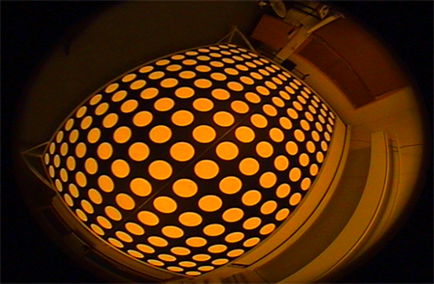Figure 5. Pictures taken with fish-eye lenses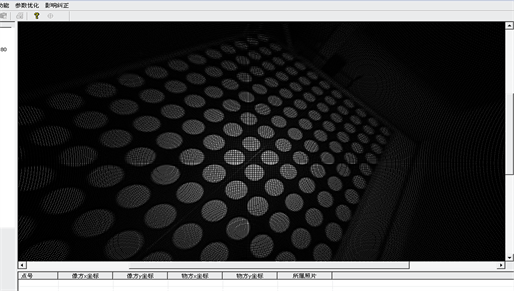Figure 6. Corrected image of one of the channels

  英向华, 胡占义. 一种基于球面透视投影约束的鱼眼镜头校正方法[J]. 计算机学报, 2003, 26(12): 1702-1708.  邱志强, 等. 用神经网络变易有效焦距的摄像机标定法[J]. 国防科技大学学报, 2002, 24(5): 16-19.  Zhang, Z. (2000) A Flexible New Technique for Camera Calibration. IEEE Transactions on Pattern Analysis and Machine Intelligence, 22, 1330-1334. https://doi.org/10.1109/34.888718  詹总谦. 基于纯平液晶显示器的相机标定方法与应用研究[D]: [博士学位论文]. 武汉: 武汉大学, 2006.  张永军, 张祖勋, 张剑清. 利用二维DLT及光束法平差进行数字摄像机标定[J]. 武汉大学学报(信息科学版), 2002, 27(6): 566-571.  Kannala, J. and Brandt, S.S. (2006) A Generic Camera Model and Calibration Method for Conventional, Wide-Angle, and Fish-Eye Lenses. IEEE Transactions on Pattern Analysis and Machine Intelligence, 28, 1335-1340. https://doi.org/10.1109/TPAMI.2006.153  Thirthala, S. and Pollefeys, M. (2005) Multi-View Geometry of 1D Radial Cameras and Its Application to Omnidirectional Camera Calibration. Tenth IEEE International Conference on Computer Vision, Beijing, 17-21 October 2005, 1539-1546. https://doi.org/10.1109/ICCV.2005.158  Barreto, J.P. and Daniilidis, K. (2005) Fundamental Matrix for Cameras with Radial Distortion. Tenth IEEE International Conference on Computer Vision, Beijing, 17-21 October 2005, 625-632. https://doi.org/10.1109/ICCV.2005.103  Kannala, J. and Brandt, S. (2004) A Generic Camera Calibration Method for Fish-Eye Lenses. Proceedings of the 17th International Conference on Pattern Recognition, Cambridge, 26-26 August 2004, 10-13. https://doi.org/10.1109/ICPR.2004.1333993